# Electronics and Communication Engineering - Exam Questions Papers

11.

Radiation resistance of an antenna is 54 Ω and loss resistance is 6 Ω. If antenna has power gain of 10, then directivity is:

 A. 9 B. 11.11 C. data not sufficient D. 10

Explanation:

Efficiency of antenna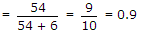Power gain = 10

Thus directivity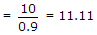.

12.

If the power spectral density of stationary random process is a sine-squared function of frequency, the shape of its autocorrelation is

 A.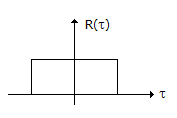B.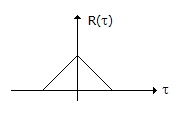C.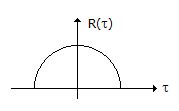D.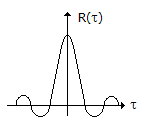Explanation:

Since autocorrelation function and power spectral density bears a Fourier transform relation, then since required in frequency domain will five rectangular convolutions in time domain thus it is triangular function.

13.

The inverse of given Laplace transform is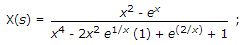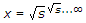A. sin t B. cos t C. et D. e2t

Explanation:s = x2 - ex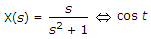x(t) = cos t.

14.

Consider the amplitude modulated (AM) signal Ac cos ωct + 2 cos ωmt cos ωct For demodulating the signal using envelope detector, the minimum value of Ac should be

 A. 2 B. 1 C. 0.5 D. 0

Explanation:

AC cos ωct + 2 cos ωmt cos ωct

AC cosωct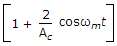for envelope detection μ < 1 ⇒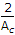< 1 ⇒ Ac should be at least-2.

15.

In a 4 bit weighted resistor D/A converter, the resistor value corresponding to LSB is 32 kΩ. The resistor value corresponding to MSB will be

 A. 32 KΩ B. 16 KΩ C. 8 KΩ D. 4 KΩ

Explanation:

2n - 1 R = 32 K&Omeg;

n = 4

⇒ 8R = 32

R = 4 KΩ.